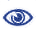# Prague Stringology Conference 2019

Computing Maximal Palindromes and Distinct Palindromes in a Trie

 Abstract: It is known that all maximal palindromes of a given string T of length n can be computed in O(n) time by Manacher's algorithm [J. ACM '75]. Also, all distinct palindromes in T can be computed in O(n) time [Groult et al., Inf. Process. Lett. 2010]. In this paper, we consider the problem of computing maximal palindromes and distinct palindromes of a given trie T (i.e. rooted edge-labeled tree). A trie is a natural generalization of a string which can be seen as a single path tree. We propose algorithms to compute all maximal palindromes and all distinct palindromes in T in O(N log h) time and O(N) space, where N is the number of edges in T and h is the height of T. To our knowledge these are the first sub-quadratic time solutions to these problems.

 Download paper:PostScript PDF BibTeX reference
 Download presentation: